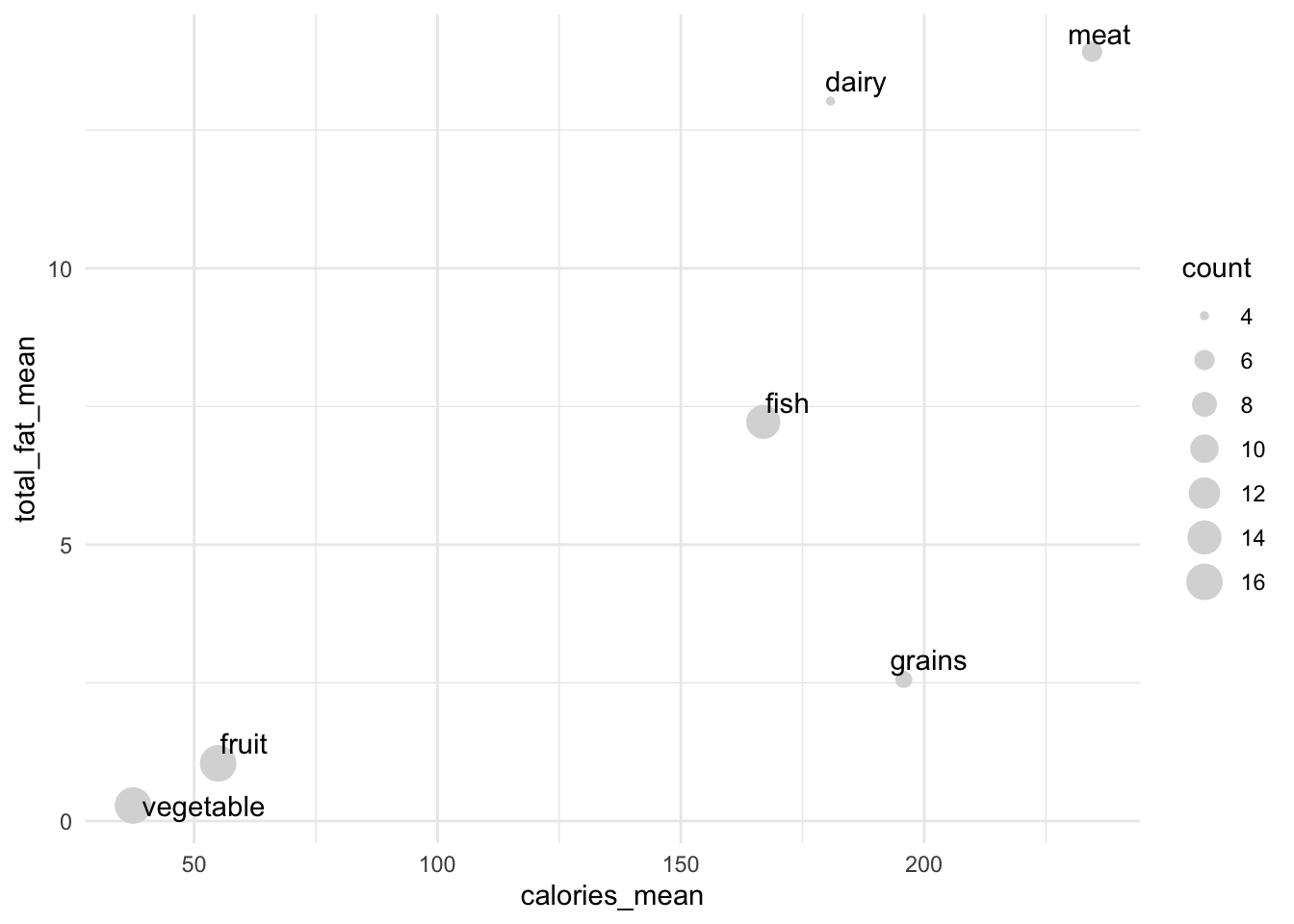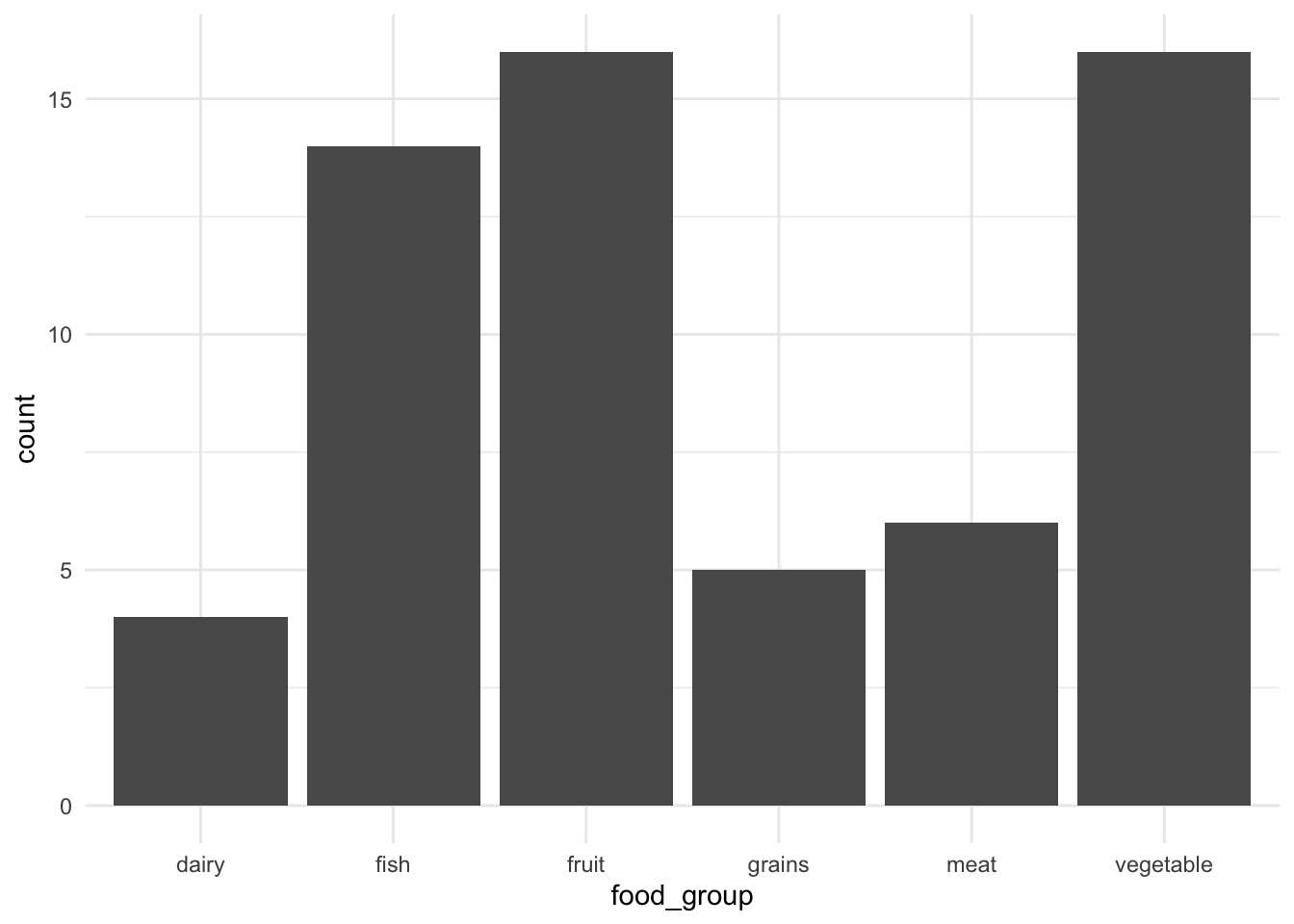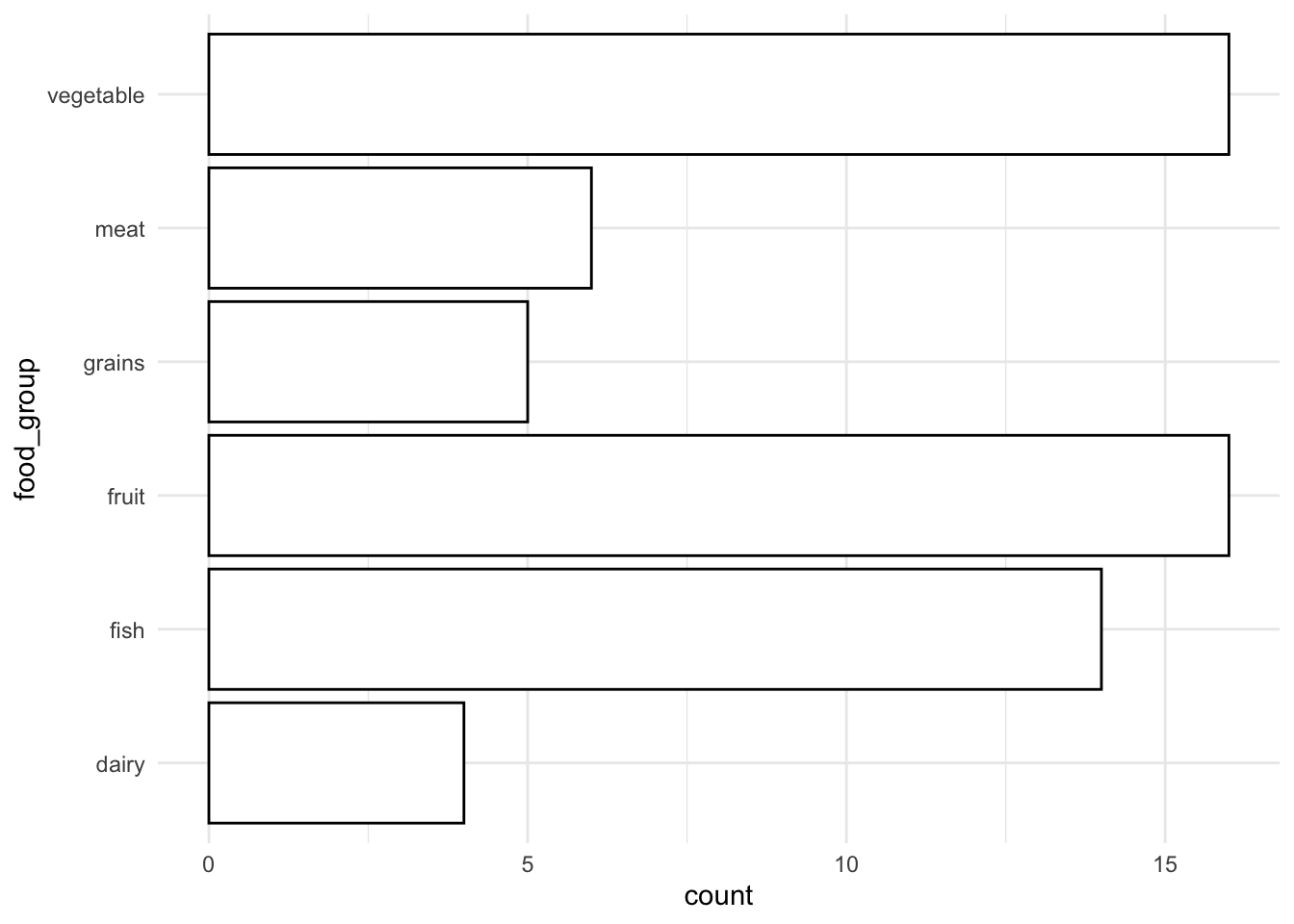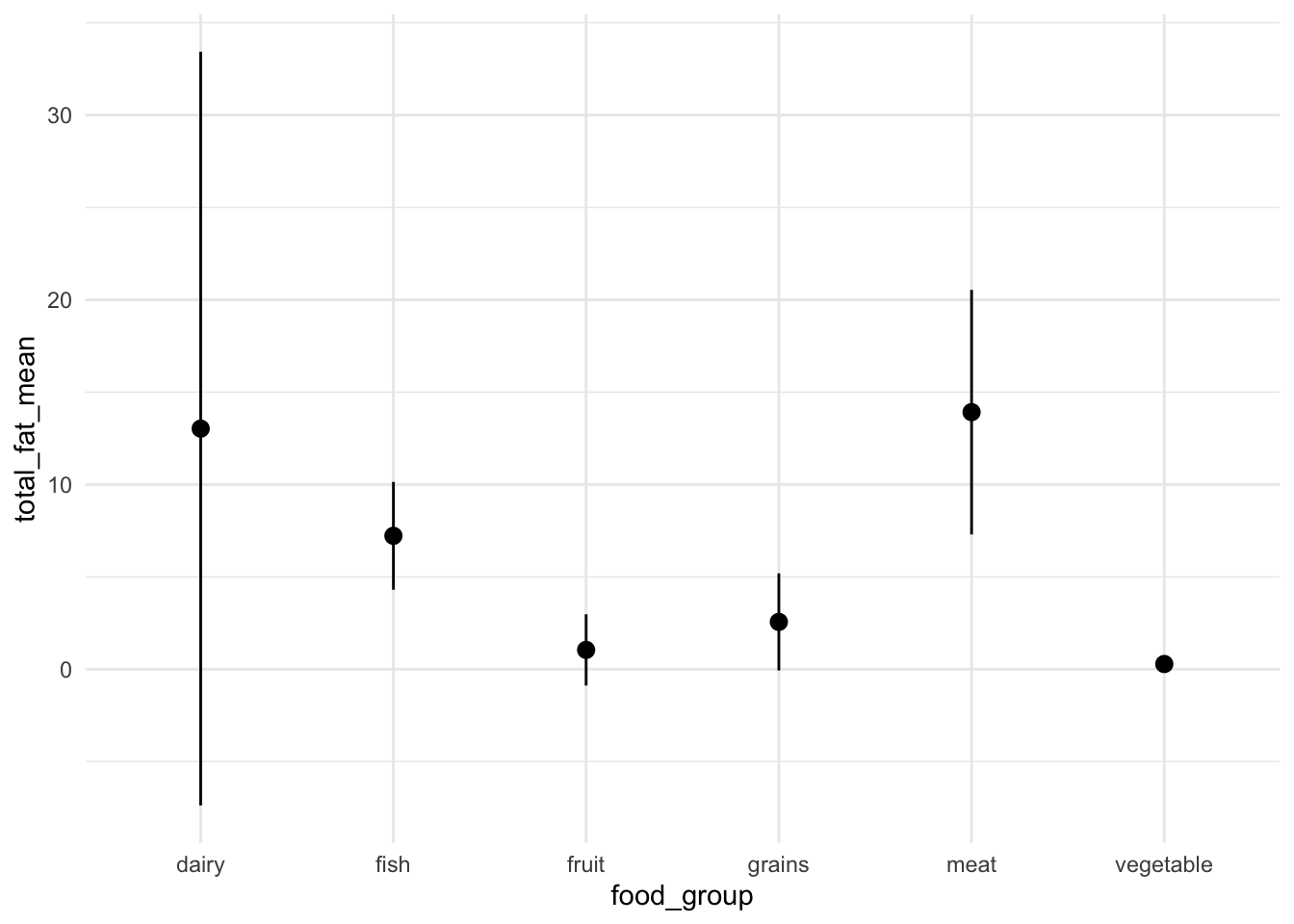## Getting Started

Before running this notebook, select “Session > Restart R and Clear Output” in the menu above to start a new R session. This will clear any old data sets and give us a blank slate to start with.

After starting a new session, run the following code chunk to load the libraries and data that we will be working with today.

I have set the options `include=FALSE` and `message=FALSE` to avoid cluttering the solutions with all the output from this code.

# Summarizing Data

## The summarize verb

In the previous notebook we introduced the concept of data verbs. Four useful examples were shown: `slice` and `filter` for taking a subset of rows, `select` for taking a subset of columns, and `arrange` for reordering a data set’s rows. In this notebook we discuss another important verb, `summarize` that collapses a data frame by using summary functions. Using this verb is slightly more involved because we have to explain exactly how the data should be summarized. We will introduce several helper functions to make this process slightly easier.

Before describing the syntax for the summarize function, let’s start with an example. Here, we summarize our food data set by indicating the mean (average) value of the sugar variable across the entire data set:

``````food %>%
summarize(sm_mean(sugar))``````
``````## # A tibble: 1 x 1
##   sugar_mean
##        <dbl>
## 1       3.42``````

Here we used the function `sm_mean` inside of the function `summarize` to produce the output. We specified which variable to compute the mean of by giving its name inside of the `sm_mean` function. The results shows us that the average amount of sugar in a 100g portion of all of the foods is 3.419g.

In order to compute multiple summaries at once, we can pass multiple functions together are once. For example, here we compute the mean value of three nutritional measurements:

``````food %>%
summarize(sm_mean(sugar), sm_mean(calories), sm_mean(vitamin_a))``````
``````## # A tibble: 1 x 3
##   sugar_mean calories_mean vitamin_a_mean
##        <dbl>         <dbl>          <dbl>
## 1       3.42          114.           16.1``````

Notice that R creates a new data set and intelligently chooses the variable names. There are a number of other useful summary functions that work similarly, such as `sm_min`, `sm_max`, `sm_sum`, and `sm_sd` (standard deviation).

## Multiple output values

Some summary functions return multiple columns for a given variable. For example, `sm_quartiles` gives the five-number summary of a variable: its minimum value, the first quartile (25th percentile), the median (50th percentile), the third quartile (75th percentile), and the maximum value. As with the other summary functions, smart variable names are automatically created in R:

``````food %>%
summarize(sm_quartiles(calories))``````
``````## # A tibble: 1 x 5
##   calories_min calories_q1 calories_median calories_q3 calories_max
##          <dbl>       <dbl>           <dbl>       <dbl>        <dbl>
## 1           12          34              87         171          389``````

Functions such as `sm_deciles` and `sm_percentiles` give a similar output, but with additional cutoff values. These can be useful in trying to describe the distribution of numeric variables in large data sets.

The final group of summary functions here provide confidence intervals. These provide the mean of a variable as well as an upper and lower bound for the mean using properties from statistical inference. Here, for example, is how we use the `sm_mean_cl_normal` to produce a confidence interval for the mean of the calories variable:

``````food %>%
summarize(sm_mean_cl_normal(calories), sm_count())``````
``````## # A tibble: 1 x 4
##   calories_mean calories_ci_min calories_ci_max count
##           <dbl>           <dbl>           <dbl> <int>
## 1          114.            90.0            137.    61``````

## Grouped summaries

Summarizing the data set to a single row can be useful for understanding the general trends in a data set or highlighting outliers. However, the real power of the summary function comes when we pair it with grouped manipulations. This will allow us to produce summaries within one or more grouping variables in our data set.

When we use the `group_by` function, subsequent uses of the `summarize` function will produce a summary that describes the properties of variables within the variable used for grouping. The variable name(s) placed inside of the `group_by` function indicate which variable(s) should be used for the groups. For example, here we compute the mean number of calories of each food group:

``````food %>%
group_by(food_group) %>%
summarize(sm_mean(calories))``````
``````## # A tibble: 6 x 2
##   food_group calories_mean
##   <chr>              <dbl>
## 1 dairy              181.
## 2 fish               167.
## 3 fruit               54.9
## 4 grains             196.
## 5 meat               234.
## 6 vegetable           37.4``````

Notice that the output data set contains a column for the grouping variable (`food_group`) and the summarized variable (`calories_mean`). The summarized variable name is exactly the same as the non-grouped version and the final line of code looks exactly the same as before. However, the output data set now contains six rows, one for each food group.

Any summarization function that can be used for an ungrouped data set can also be used for a grouped data set. Also, as before, we can put multiple summary functions together to obtain different measurements of each group.

``````food %>%
group_by(food_group) %>%
summarize(sm_mean(calories), sm_mean(total_fat))``````
``````## # A tibble: 6 x 3
##   food_group calories_mean total_fat_mean
##   <chr>              <dbl>          <dbl>
## 1 dairy              181.          13.0
## 2 fish               167.           7.22
## 3 fruit               54.9          1.04
## 4 grains             196.           2.56
## 5 meat               234.          13.9
## 6 vegetable           37.4          0.281``````

Notice that the automatically produced variable names should make it clear which column corresponds to each summary function.

## More summary functions

There are several additional summary functions that will be useful for analyzing data. The function `sm_count` takes no arguments and returns a variable called `count` that counts the total number of rows in the data set:

``````food %>%
group_by(food_group) %>%
summarize(sm_count())``````
``````## # A tibble: 6 x 2
##   food_group count
##   <chr>      <int>
## 1 dairy          4
## 2 fish          14
## 3 fruit         16
## 4 grains         5
## 5 meat           6
## 6 vegetable     16``````

This tells us how many times each type of food group occurs in the data set. Similarly, the function `sm_na_count` tells us how many values of a variable are missing:

``````food %>%
group_by(food_group) %>%
summarize(sm_count(), sm_na_count(calories))``````
``````## # A tibble: 6 x 3
##   food_group count calories_na_count
##   <chr>      <int>             <int>
## 1 dairy          4                 0
## 2 fish          14                 0
## 3 fruit         16                 0
## 4 grains         5                 0
## 5 meat           6                 0
## 6 vegetable     16                 0``````

In this case there are no missing values for the `calories` variable.

The summary function `sm_paste` collapses all of the values in a character variable. For example, applying this summary it to the `item` category after grouping by color, we can see all of the foods in the data set associated with a specific color:

``````food %>%
group_by(color) %>%
summarize(sm_paste(item))``````
``````## # A tibble: 8 x 2
##   color  item_paste
##   <chr>  <chr>
## 1 brown  Chickpea; Mushroom; Oat; Quinoa; Brown Rice
## 2 green  Asparagus; Avocado; String Bean; Bell Pepper; Broccoli; Cabbage; Ce…
## 3 orange Cantaloupe; Carrot; Orange; Sweet Potato; Tangerine
## 4 pink   Grapefruit; Peach; Salmon; Shrimp
## 5 purple Grape; Plum
## 6 red    Apple; Beef; Crab; Duck; Lamb; Lobster; Strawberry; Tomato; Tuna
## 7 white  Catfish; Cauliflower; Chicken; Clam; Cod; Flounder; Halibut; Haddoc…
## 8 yellow Banana; Cheese; Corn; Lemon; Pineapple``````

Do the foods correspond to the colors that you would expect?

Finally, note that it is possible to define your own summary functions using other R functions. To do this, we have to specify the name of the new variable explicitly. For example, here is an alternative way of computing the mean of the amount of Vitamin A within each food color:

``````food %>%
group_by(color) %>%
summarize(avg_vitamin_a = mean(vitamin_a)) %>%
arrange(desc(avg_vitamin_a))``````
``````## # A tibble: 8 x 2
##   color  avg_vitamin_a
##   <chr>          <dbl>
## 1 orange        141.
## 2 green          11.1
## 3 pink            8.75
## 4 yellow          4.4
## 5 purple          4
## 6 red             2.78
## 7 white           2.63
## 8 brown           0``````

As we saw in the previous notebook, orange foods have a very high amount of Vitamin A compared to the other food colors.

## Geometries for summaries

We can use summarized data sets to produce new data visualizations. For example, consider summarizing the average number of calories, average total fat, and number of items in each food groups. We can take this data and construct a scatter plot that shows the average fat and calories of each food group, along with informative labels. Here’s the code to make this visualization:

``````food %>%
group_by(food_group) %>%
summarize(sm_mean(calories), sm_mean(total_fat), sm_count()) %>%
ggplot(aes(calories_mean, total_fat_mean)) +
geom_point(aes(size = count), color = "grey85") +
geom_text_repel(aes(label = food_group))``````If this seems complex, don’t worry! We are just putting together elements that we have already covered, but it takes some practice before it becomes natural.

Scatterplots are often useful for displaying summarized information. There are two additional `geom` types that often are useful specifically for the case of summarized data sets.

If we want to create a bar plot, where the heights of the bars as given by a column in the data set, we can use the `geom_col` layer type. For this, assign a categorical variable to the `x`-aesthetic and the count variable to the `y`-aesthetic. For example, here is a bar plot showing the number of items in each food group:

``````food %>%
group_by(food_group) %>%
summarize(sm_count()) %>%
ggplot() +
geom_col(aes(x = food_group, y = count))``````There are two specific things to keep in mind with the `geom_col` layer. First, there are two color-related `aes` categories: the border of the bars (`color`) and the color used to shade the inside of the bars (`fill`). We can change these exactly as we did with the single color value used with scatter plots. Also, if we want to produce a bar plot with horizontal bars, this can be done by adding the special layer `coord_flip()` at the end of the plotting command.

``````food %>%
group_by(food_group) %>%
summarize(sm_count()) %>%
ggplot(aes(x = food_group, y = count)) +
geom_col(color = "black", fill = "white") +
coord_flip()``````I find that using a white fill color and a black border is often a good-looking starting point. Also, you will notice that making the bars horizontal will make it easier to read the category names when there are a larger number of categories.

There is also a specific geometry that is useful when visualizing confidence intervals called `geom_pointrange`. It requires a categorical `x`-aesthetic, a numeric `y`-aesthetic, and two additional numeric aesthetics: `ymin` and `ymax`. This produced a visual confidence interval from the minimum value to the maximum value, with the middle value shown by a solid point:

``````food %>%
group_by(food_group) %>%
summarize(sm_mean_cl_normal(total_fat)) %>%
ggplot() +
geom_pointrange(aes(
x = food_group,
y = total_fat_mean,
ymin = total_fat_ci_min,
ymax = total_fat_ci_max
))``````Here, we see that vegetables have a low amount of total fat, meats have a relatively large amount of fat, and the confidence interval for dairy products is very large (in this case, it is because there are not many dairy products in the data set). As with the bar plot, we can draw the confidence intervals horizontally by adding a `coord_flip()` layer to the plot.

## Multiple groups

As mentioned above, it is possible to group a data set by multiple variables. To do this, we can provide additional variables to the `group_by` function separated by commas. For example, we could group the food data set into food group and color, and summarize each combination of the two:

``````food %>%
group_by(food_group, color) %>%
summarize(sm_count(), sm_mean(calories))``````
``````## # A tibble: 21 x 4
## # Groups:   food_group 
##    food_group color  count calories_mean
##    <chr>      <chr>  <int>         <dbl>
##  1 dairy      white      3         124.
##  2 dairy      yellow     1         350
##  3 fish       pink       2         158.
##  4 fish       red        3         112.
##  5 fish       white      9         187.
##  6 fruit      green      4          77.2
##  7 fruit      orange     3          44.7
##  8 fruit      pink       2          35.5
##  9 fruit      purple     2          57.5
## 10 fruit      red        2          42
## # … with 11 more rows``````

Notice that now there is one row for each combination of the two groups. However, there is no row for combinations that do not exist. So, there is no row for pink dairy products nor for white fruit. Examples of several common uses for multiple groups are given in the exercises.

# Practice

## Load Datasets

We will work with the largest cities datasets:

``cities <- read_csv(file.path("data", "largest_cities.csv"))``

We will also work with the entire U.S. cities dataset:

``us <- read_csv(file.path("data", "us_city_population.csv"))``

Please refer to the previous notebooks for more information about these data sets.

## Summary Statistics

In the code block below, using the `summarize` function to compute the mean city population (`city_pop`) in the `cities` dataset.

``````cities %>%
summarize(sm_mean(city_pop))``````
``````## # A tibble: 1 x 1
##   city_pop_mean
##           <dbl>
## 1          7.80``````

Now, compute the number of missing values for the city population variable (`city_pop`) using the function `sm_na_count`.

``````cities %>%
summarize(sm_na_count(city_pop))``````
``````## # A tibble: 1 x 1
##   city_pop_na_count
##               <int>
## 1                 7``````

Notice that these missing values were ignored in the calculation of the average value in the previous calculation.

Now, compute the quartiles of the city area variable:

``````cities %>%
summarize(sm_quartiles(city_pop))``````
``````## # A tibble: 1 x 5
##   city_pop_min city_pop_q1 city_pop_median city_pop_q3 city_pop_max
##          <dbl>       <dbl>           <dbl>       <dbl>        <dbl>
## 1        0.236        2.73            7.36        10.7         30.2``````

What is the 25th percentile of city sizes in the dataset? Answer: 2.76 million.

Let’s compute multiple summaries in one command. Below, using the summarize function to calculate the average value of each of the four population variables.

``````cities %>%
summarize(
sm_mean(population),
sm_mean(city_pop),
sm_mean(metro_pop),
sm_mean(urban_pop)
)``````
``````## # A tibble: 1 x 4
##   population_mean city_pop_mean metro_pop_mean urban_pop_mean
##             <dbl>         <dbl>          <dbl>          <dbl>
## 1            10.5          7.80           12.9           12.7``````

Which of the population counts is on average the smallest? Which is on average the largest? Answer: City Population is the smallest and metro population is the largest.

The correlation between two variables indicates the “strength and direction of a linear relationship” between them. Here, use the summarize function to compute the correlation between the city population and city area using the summary command `sm_cor()`:

``````cities %>%
summarize(sm_cor(city_pop, city_area))``````
``````## # A tibble: 1 x 1
##   city_pop_city_area_cor
##                    <dbl>
## 1                  0.495``````

## Grouped Summaries

Let’s now try to use grouped summarize functions. There is a variable in the `cities` dataset called `city_definition`. It describes the kind of administrative structure given to each city. Using a grouped summary, in the code below tabulate how many times each city definition is used in the dataset. Arrange the data in decreasing order from the most common to least common definition.

``````cities %>%
group_by(city_definition) %>%
summarize(sm_count()) %>%
arrange(desc(count))``````
``````## # A tibble: 27 x 2
##    city_definition           count
##    <chr>                     <int>
##  1 Municipality                 20
##  2 City (sub-provincial)        14
##  3 City                          8
##  4 Other                         6
##  5 Capital city                  3
##  6 Designated city               3
##  7 Metropolitan municipality     3
##  8 Urban governorate             3
##  9 Federal city                  2
## 10 Metropolitan city             2
## # … with 17 more rows``````

What city type is the most common in the dataset? Answer: Minicipality.

Now, in the code below group by continent and paste together the city names (`name`).

``````cities %>%
group_by(continent) %>%
summarize(sm_paste(name))``````
``````## # A tibble: 5 x 2
##   continent     name_paste
##   <chr>         <chr>
## 1 Africa        Cairo; Lagos; Kinshasa; Luanda; Dar es Salaam; Khartoum; Joh…
## 2 Asia          Tokyo; Delhi; Shanghai; Mumbai; Beijing; Dhaka; Osaka; Karac…
## 3 Europe        Istanbul; Moscow; Paris; London; Madrid; Barcelona; Saint Pe…
## 4 North America Mexico City; New York City; Los Angeles; Chicago; Houston; D…
## 5 South America São Paulo; Buenos Aires; Rio de Janeiro; Bogotá; Lima; Santi…``````

You will probably have to scroll over to see the results.

Finally, in the code below group by continent, count the number of cities in each continent, and pass this to a plot with a `geom_col` layer to visualize the number of cities on each continent.

``````cities %>%
group_by(continent) %>%
summarize(sm_count()) %>%
ggplot(aes(continent, count)) +
geom_col()``````